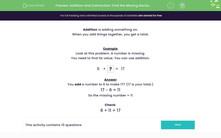# Find the Missing Number Using Inverse Operation

In this activity, students will solve number problems using addition or subtraction to find the missing value (0-20).Key stage:  KS 1

Curriculum topic:   Number: Addition and Subtraction

Curriculum subtopic:   Solve Add/Subtract Problems to 20

Popular topics:   Subtraction worksheets, Addition worksheets

Difficulty level:#### Worksheet Overview

When you add things together, you get a total.

Addition and subtraction are linked together and here we are going to look at using subtraction to solve an addition question.

Example

Look at this problem. We need to work out what the missing number is.

 6 + ? = 17

We are starting on 6 and adding something on to make 17.

One way we can work this out is by using the inverse, which is subtraction.

Using the two numbers that we already have in this number sentence, we can move them around to solve the question using subtraction.

We put the biggest number in the number sentence first, which is 17.

The other number left is 6, so we use this number to subtract from 17. Here is our subtraction number sentence:

17 - 6 =

We can use this subtraction number sentence to find the missing number.

Start on 17 and count back 6.

17, 16, 15, 14, 13, 12, 11

This leaves us with 11

17 - 6 = 11

Now we have worked out the missing number we can put it back into the addition number sentence and check it works!

 6 + 11 = 17

Let's have a go at some questions now.### What is EdPlace?

We're your National Curriculum aligned online education content provider helping each child succeed in English, maths and science from year 1 to GCSE. With an EdPlace account you’ll be able to track and measure progress, helping each child achieve their best. We build confidence and attainment by personalising each child’s learning at a level that suits them.

Get started